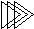Electronic Design with Excel
 Topics | VBA Basics | Back to eCircuit Center

VBA BASICS

WHAT IS VBA?

Microsoft Excel includes a built in version of the programming language BASIC. Specifically, VBA (Visual Basic with Applications) allows users to customize Excel spreadsheets (add special functions, buttons, print outs, reports) for various applications (engineering, sciences, payroll, purchasing, accounting, etc.)

I'm so glad I discovered VBA! Its a great tool if you're interested in electronics design and analysis! While Excel is a workhorse of the the electronics industry, it's not visually friendly when it comes to displaying equations or working with algorithms. But, VBA let's you write equations with meaningful variables. And to boot, you only have to write the equation once. Then simply call the function where you need it. Made a mistake? You only have to correct it once in the function.

VBA EXAMPLE

To get a first view at VBA, we'll use a simple resistor divider. The input voltage vs, gets divided by R1 and R2 to produce an output voltage
vo = vs * R2 / (R1 + R2).

 Input Signal Vs 10 Resistor Divider R1 10000 R2 20000 Gain and Output of Divider K Rdiv 0.667 Vo 6.667

The red cell holds the function call

=K_Rdiv1(B17,B18)

What does the VBA code look like? Hit ALT-F11 and double click on the Modules > Modules1 in the VBA Project window. This opens the code window for this module.

 Function K_Rdiv1(R1, R2)   ' Gain of resistor divider   K_Rdiv1 = R2 / (R2 + R1)End Function

INSIDE THE FUNCTION

What makes up this function? The first and last lines bookend the function to let the VBA compiler know which statements make up this function. The text following the ' is ignored by the compiler. This is the place for your comments! Take some time to note what you did and why. For more complex functions, you'll be desperately scanning these comments later when you're reusing or debugging some code and trying to figure out - what was I thinking?

The meat of the function is the actual calculation of the resistor attenuation. Note that the result is placed in a variable with the same name as the function, K_Rdiv1. This is no accident. When executed, the value of K_Rdiv1 gets placed into cell from which it was called. Cool!

Okay, this ultra simple function did not showcase some of VBA's more advanced capabilities. But, it served our introductory purposes. There will be more interesting functions to challenge you coming around the bend.

The best part of VBA is that it lets you play with the code. Once you see some code, jump right in and start messing with it or write a new function altogether. Just copy the existing code to a space just below the original. Then start modifying the name and calculations. Here's a few ideas you can try:

1. Write a function to calculate the parallel combo of two resistors. You can name the function something like "Function R_Par(R1, R2)".
2. Make a function to calculate the non-inverting gain of an op amp.